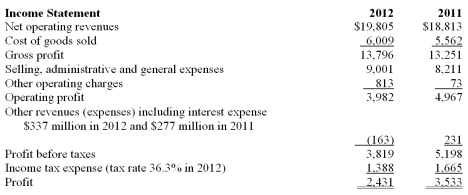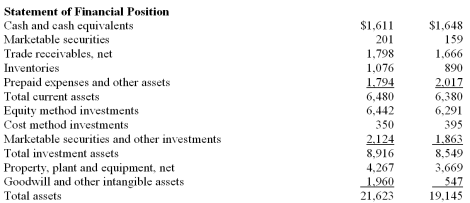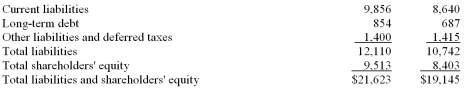/
/
/
41. Lyceum Co. reported profit of \$8.3 million, interest expense
Not my Question
Flag Content

# Question : 41. Lyceum Co. reported profit of \$8.3 million, interest expense : 2048908

41. Lyceum Co. reported profit of \$8.3 million, interest expense of \$.5 million and they are in a 30% tax rate bracket. Their average total assets are \$65.8 million and average shareholders' equity is \$48.6 million. What is Lyceum's financial leverage advantage or disadvantage?

A. 3.7%

B. 3.9%

C. 4.0%

D. 4.7%

The following information was taken from the financial statements of C Co for the years 2012 and 2011:42. Calculate C Co's current ratio for 2012 and 2011 respectively.

A. .54 and .59

B. .60 and .70

C. .66 and .74

D. .63 and .72

43. Calculate C Co's quick ratio for 2020 and 2011 respectively.

A. .30 and .32

B. .37 and .40

C. .55 and .64

D. .56 and .54

44. Calculate C Co's receivables turnover ratio and the days' sales in receivables for 2012.

A. 11.43 times and 31.9 days

B. 11.02 times and 33.1 days

C. 11.15 times and 32.7 days

D. 3.47 times and 105.2 days

45. Calculate C Co's inventory turnover ratio and the days' sales in inventory for 2012.

A. 5.89 times and 62.0 days

B. 18.41 times and 19.8 days

C. 5.58 times and 65.4 days

D. 6.11 times and 59.7 days

## Solution 5 (1 Ratings )

Solved
Finance 2 Days Ago 10 Views